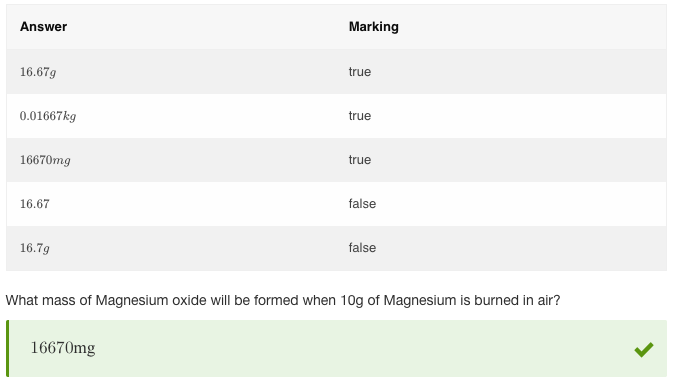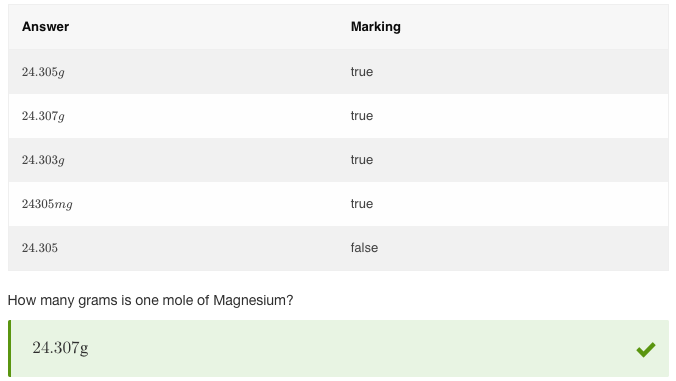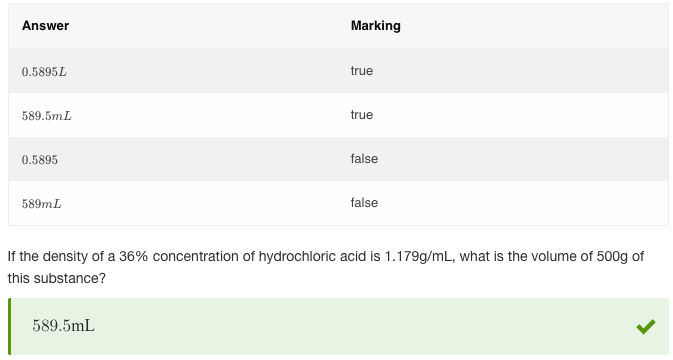# equivValue Scoring Method

## Overview

equivValue compares numerical values that may be represented in different ways, such as 1L = 1000mL. equivValue will evaluate the expression to a numerical form for comparison. This means that as long as the value given by the student is equal to the result the author was expecting, the question will validate as correct.

Use equivValue if you are expecting a numeric result or if using units of measure.

• Tolerance
Ignores the order of expressions. E.g. both x+1 and 1+x will be considered correct. If this is not enabled, equivLiteral will not accept the correct response specified by the Author in a different order, even if it is an acceptable answer.

• Inverse result
Enabling this means that the value specified in the Value field will not be accepted as the correct answer. It is a useful way of excluding very specific answers from validation. Use case: Students are asked to enter something symbolically equivalent to `(x+2)^2`, however you don't want them to enter the same expression and score points for such response. In this case, adding an additional scoring method equivLiteral and enabling Inverse Result will exclude (x+2)^2 from correct response options. See examples in the Combining Methods section below.
• Significant decimal places
This option defines how many decimal places can be allowed in students responses. Default and maximum value is 10.

## Examples

Example 1 - Basic scoring with units

As long as the student's answer has the same value as the valid response, the question will be correct. Answers can be given in different units.Figure 1: Basic scoring with units

Example 2 - Tolerance Plus/Minus

In this example, a tolerance of 0.002 has been added.Figure 2: Tolerance Plus/Minus

## Combining methods

Example 3 - equivValue + equivLiteral, restricting unit types

Combining equivValue with isUnit is a good method of narrowing down possible response options if needed. In this example, the answer should be given in either L or mL. Listing L and mL in isUnit settings ensures that only correct vales given in these units of measurement will be valid.Figure 3: Combining equivValue + equivLiteral, restricting unit types